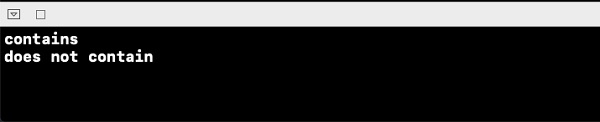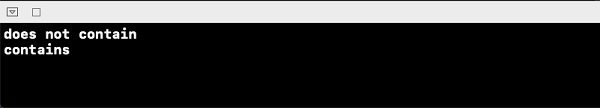# Check if string contains another string in Swift

To check if a string contains another string in swift, we’ll need two different strings. One string that we have to check if it consists another string.

Let us say the string we want to check is “point” and the whole string is “TutorialsPoint” and another string is “one two three”. Let’s check with both these string in the playground.

We can do this in two ways as shown below. Let’s start by creating three different strings.

var CompleteStr1 = "Tutorials point"
var completeStr2 = "one two three"
var stringToCheck = "point"

## Method One

In this method we’ll use the .contains method of Strings to check if there is a string within another string, it returns true if it exists, otherwise, it returns false.

if CompleteStr1.contains(stringToCheck) {
print("contains")
} else {
print("does not contain")
}

## Method Two

In this method we’ll check the range of a string if the range is nil, it means that the string we are checking for, does not exist. Otherwise, it means that string exists.

if completeStr2.range(of: stringToCheck) != nil {
print("contains")
} else {
print("does not contain")
}

When we run the above code, we get the output as shown below.Similarly, let’s try these methods with one more example.

var Str1 = "12312$$33@" var Str2 = "%%" var Str3 = "$$"
if Str1.contains(Str2) {
print("contains")
} else {
print("does not contain")
}
if Str1.range(of: Str3) != nil {
print("contains")
} else {
print("does not contain")
}

This produces the result as shown below.